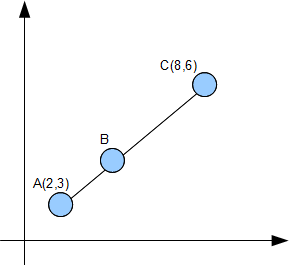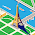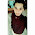### Coordinate Geometry Part II

Example 1

A typical coordinate geometry question from the O LevelsGiven that AC = 3AB, find the coordinates of point B.

Remarks:
A lot of students will try to use the distance formula to form simultaneous equations in x and y to find the coordinates of B. This is a very long and tedious method. A much simpler method is to use ratio and proportion to find the coordinates of B

To find the x coordinate of BWe can use the same method to find the y coordinate of point BTherefore the coordinates of point B is (4,4)

1.Hey, this is a great post, thank you so much for sharing. I’m looking forward to coming back to your site for more great information. Keep up the good work! : )

2.No maths phobia can stop you from becoming an excellent mathematician if you practise Vedic maths after learning it at Vedic maths classes in Delhi.
http://supremedissertation.co.uk/